• 各种颜色小球 原理讲解 霍夫圆变换：的基本原理和上个教程中提到的霍夫线变换类似, 只是点对应的二维极径极角空间被三维的圆心点x,yx,yx,y还有半径rrr空间取代。 原理：从平面坐标圆上的点到极坐标转换的三个参数C...
关键字
模糊滤波霍夫圆检测框出小球文字绘制
所需配件
powersensor传感器各种颜色小球
原理讲解
霍夫圆变换：的基本原理和上个教程中提到的霍夫线变换类似, 只是点对应的二维极径极角空间被三维的圆心点

x

,

y

x,y

还有半径

r

r

空间取代。 原理：从平面坐标圆上的点到极坐标转换的三个参数

C

(

x

0

,

y

0

,

r

)

C(x_0,y_0,r)

其中

x

0

,

y

0

x_0,y_0

是圆心，

r

r

取一固定值时扫描360度，

x

,

y

x,y

跟着变化， 若多个边缘点对应的三维空间曲线交于一点,则他们在共同圆上，在圆心处有累积最大值，也可以用同样的阈值的方法来判断一个圆是否被检测到。
相关API - HoughCircles
因为霍夫圆检测对噪声比较敏感，所以首先要对图像做滤波（比如椒盐噪声用中值滤波，其他的也可以用高斯模糊）。基于效率考虑，Opencv中实现的霍夫变换圆检测是基于图像梯度（霍夫梯度法, 也叫2-1霍夫变换(21HT)）的实现，分为两步（已封装到HoughCircles）： （1）Canny检测边缘，发现可能的圆心。圆心一定是在圆上的每个点的模向量上, 这些圆上点模向量的交点就是圆心, 霍夫梯度法的第一步就是找到这些圆心, 这样三维的累加平面就又转化为二维累加平面。 （2）基于第一步的基础上从候选圆心开始计算最佳半径大小。第二步根据所有候选中心的边缘非0像素对其的支持程度来确定半径。
函数介绍
cv2.HoughCircles(image, method, dp, minDist[, circles[, param1[, param2[,minRadius[, maxRadius]]]]]) → circles
# image,必须，原图,要求二值化后的图片；
# dp,必须，检测的缩放比例参数，如果为1，就是检测原图，为2就是缩小一半检测，影响计算效率；
# minDist，为霍夫变换检测到的圆的圆心之间的最小距离，即让我们的算法能明显区分的两个不同圆之间的最小距离。这个参数如果太小的话，多个相邻的圆可能被错误地检测成了一个重合的圆。反之，这个参数设置太大的话，某些圆就不能被检测出来了。
# param1，有默认值100。它是method设置的检测方法的对应的参数。对当前唯一的方法霍夫梯度法，它表示传递给canny边缘检测算子的高阈值，而低阈值为高阈值的一半。
# param1 , 可 选 ， 低 阈 值 ， 传 递 给 canny() 检 测 器 的 低 阈 值 ；

 cv2.putText(src, text, place, Font, Font_Size, Font_Color, Font_Overstriking)
# src，输入图像
# text，需要添加的文字
# place，左上角坐标
# Font，字体类型
# Font_Size，字体大小
# Font_Color，文字颜色
# Font_Overstriking，字体粗细

颜色标定
TakeColor取色器
TakeColor是一款轻便小巧的屏幕色彩拾取小工具。TakeColor取色器是目前为止使用感觉比较好的取色工具，可以直接使用快捷键“alt+C”就可以取色，方便快捷，可以针对上任何位置的色彩进行自定义拾取。如需要可自行从网上下载，TakeColor截图如下：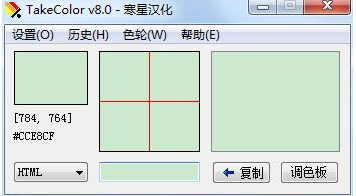TakeColor取色器功能特点：
支持鼠标点击取色和用alt+c快键捷取色，快捷方便;将屏幕上的任意一点的颜色读出来，转换成RGB和Hex形式的颜色值;具有放大镜功能，让你可以看清任何一个像素的颜色;能够显示取色点的十六位颜色值;支持调色板功能。
TakeColor取色器使用方法
双击或者右键打开takecolor取色器，看到软件主界面；我们只需要将鼠标放在屏幕目标区域上，然后同时按“Alt+C”键就可以选取到对应的颜色；如果我们需要哪种类型的色值在软件界面左下角的下拉框选择就可以了；点击复制按钮或者按Ctrl+C进行复制使用就可以了。
RGB颜色空间
RGB(red,green,blue)颜色空间最常用的用途就是显示器系统（计算机、电视机等都是采用RGB颜色空间来进行图像显示）。一般来说，电脑，电视机等是利用三个电子枪分别发射R分量，G分量，B分量的电子束，以此来激发屏幕上的RGB三种颜色的荧光粉，从而发出不同颜色、不同亮度的像素、进而组成了一幅图像；很明显，RGB颜色空间利用了物理学中的三原色叠加从而组成产生各种不同颜色的原理。在RGB颜色空间中，R、G、B三个分量的属性是独立的。也即是说，RGB颜色可以表示为（Red, Green, Blue)。其中，各个分量的数值越小，亮度越低。数值越大，亮度越高；如：（0，0，0）表示黑色，（255，255，255）表示白色；
小球颜色标定
将得到的图像进行模糊滤波处理，基本思想是用像素点邻域灰度值的中值来代替该像素的灰度值，该方法在去除脉冲噪声、椒盐噪声的同时还能保留图像的细节部分便于实现圆检测。进行圆检测过程中得到圆心坐标，将圆心作为正方形的中心计算并框出小球。在小球上选取一小块感兴趣区域，感兴趣区域(Region of Interest, ROI)的选取，一般有两种情形：1)已知ROI在图像中的位置；2)ROI在图像中的位置未知。现在我们已经知道了圆心坐标，所以是第一种情况了。直接在圆心附近选取一小块作为感兴趣区域用于后面的颜色标定（不建议取圆心一点作为判断颜色的标准，因为防止一点颜色空间发生突然跳变而检测颜色出现错误）。分离图像RGB通道，用TakeColor取色器大致判断图像RGB范围，再通过设定阈值来标定小球颜色并再框上显示出来。
参考例程
#指定编码方式
fourcc = cv2.VideoWriter_fourcc(*'MJPG')
#设置保存的文件名，图像的格式
out1 = cv2.VideoWriter('output3.avi',fourcc, 20.0, (320,240))
#小球颜色识别bgr
def colour2(img_b,img_g,img_r) :
if ((img_b>=60 and img_b<=130 )  and (img_g>=60 and img_g<=130) and (img_r>=140 and img_r<=220) ):
cv2.putText(origin,'red', (i-i,i-i), cv2.FONT_HERSHEY_PLAIN, 2.0, (0, 0, 255), 2)
elif ((img_b>=70 and img_b<=140) and (img_g>=120 and img_g<=190) and (img_r>=170 and img_r<=240) ):
cv2.putText(origin,'yellow',(i-i,i-i), cv2.FONT_HERSHEY_PLAIN, 2.0, (0, 0, 255), 2)
elif ((img_b>=70 and img_b<=140) and (img_g>=150 and img_g<=230) and (img_r>=100 and img_r<=170) ):
cv2.putText(origin,'green', (i-i,i-i), cv2.FONT_HERSHEY_PLAIN, 2.0, (0, 0, 255), 2)
elif ((img_b>=50 and img_b<=120) and (img_g>=60 and img_g<=120) and (img_r>=70 and img_r<=120) ):
cv2.putText(origin,'brown', (i-i,i-i), cv2.FONT_HERSHEY_PLAIN, 2.0, (0, 0, 255), 2)

while(True):
clear_output(wait=True)
origin = cv2.resize(imgMat, (320,240))
start = time.time()
# 转换为灰度图
img_gray = cv2.cvtColor(origin, cv2.COLOR_BGR2GRAY)
# medianBlur 平滑（模糊）处理
img_gray = cv2.medianBlur(img_gray, 7)
#圆检测
if circles is None:
pass
else:
circles = np.uint16(np.around(circles))
for i in circles[0,:]:
# 勾画正方形，origin图像、i*2是边长
cv2.rectangle(origin,(i-i,i-i),(i+i,i+i),(255,0,0), 2)
#取球心一小块区域
roi = origin[i:i , i:i+1]
#分离bgr通道
img_b = np.uint16(np.mean(roi[:,:,0]))
img_g = np.uint16(np.mean(roi[:,:,1]))
img_r = np.uint16(np.mean(roi[:,:,2]))
#判断小球颜色
colour2(img_b,img_g,img_r)

# 计 算 消 耗 时 间
end = time.time()
ps.CommonFunction.show_img_jupyter(origin)
out1.write(origin)
print(end - start)
#time.sleep(0.1)
out1.release()

结果展示
结果如图所示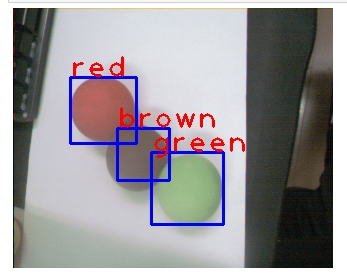框出小球并识别到三个小球颜色
小结一下
本文主要介绍了基于霍夫圆检测方法以及如何框出小球并识别颜色，霍夫变换的原理就是利用图像全局特征将边缘像素连接起来组成区域封闭边界，它将图像空间转换到参数空间，在参数空间对点进行描述，达到检测图像边缘的目的。识别小球颜色主要难点在调整RGB参数方面，利用TakeColor取色器选取参数范围，设置正确后便可以准确判断出小球颜色。
展开全文• 1.贴图用于显示材质的重要组成部分，材质是什么样子，通常由...3.经过处理后的就生成了材质，将材质添加到模型上，模型就有了材质颜色。 转载于:https://www.cnblogs.com/tthjHiroki/p/9333367.html...

1.贴图用于显示材质球的重要组成部分，材质球是什么样子，通常由贴图决定。
3.经过处理后的就生成了材质球，将材质球添加到模型上，模型就有了材质球的颜色。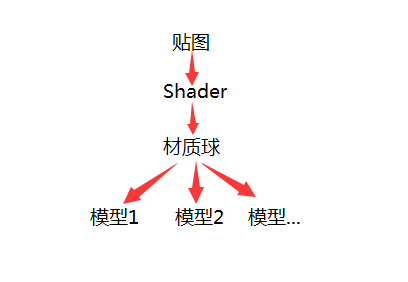转载于:https://www.cnblogs.com/tthjHiroki/p/9333367.html
展开全文• ## 朴素贝叶斯分类:原理

万次阅读 多人点赞 2019-05-29 15:29:54
贝叶斯原理是英国数学家托马斯·贝叶斯提出的。贝叶斯是个很神奇的人，他的经历类似梵高。生前没有得到重视，死后，他写的一篇关于归纳推理的论文被朋友翻了出来，并发表了。这一发表不要紧，结果这篇论文的思想直接...
贝叶斯原理是英国数学家托马斯·贝叶斯提出的。贝叶斯是个很神奇的人，他的经历类似梵高。生前没有得到重视，死后，他写的一篇关于归纳推理的论文被朋友翻了出来，并发表了。这一发表不要紧，结果这篇论文的思想直接影响了接下来两个多世纪的统计学，是科学史上著名的论文之一。
贝叶斯原理
贝叶斯为了解决一个叫“逆向概率”问题写了一篇文章，尝试解答在没有太多可靠证据的情况下，怎样做出更符合数学逻辑的推测。 什么是“逆向概率”呢？ 所谓“逆向概率”是相对“正向概率”而言。正向概率的问题很容易理解，比如我们已经知道袋子里面有 N 个球，不是黑球就是白球，其中 M 个是黑球，那么把手伸进去摸一个球，就能知道摸出黑球的概率是多少。但这种情况往往是上帝视角，即了解了事情的全貌再做判断。

一个袋子里有10个球，其中6个黑球，4个白球；那么随机抓一个黑球的概率是0.6！

在现实生活中，我们很难知道事情的全貌。贝叶斯则从实际场景出发，提了一个问题：如果我们事先不知道袋子里面黑球和白球的比例，而是通过我们摸出来的球的颜色，能判断出袋子里面黑白球的比例么？
正是这样的一个问题，影响了接下来近 200 年的统计学理论。 这是因为，贝叶斯原理与其他统计学推断方法截然不同，它是建立在主观判断的基础上：在我们不了解所有客观事实的情况下，同样可以先估计一个值，然后根据实际结果不断进行修正。 假设有一种病叫做“贝叶死”，它的发病率是万分之一，现有一种测试可以检验一个人是否得病的准确率是 99.9%，它的误报率是 0.1%，那么现在的问题是，如果一个人被查出来患有“叶贝死”，实际上患有的可能性有多大？

问题分析：随机拉一个人进行检查，误报率是0.1%。那么如果一个人被检查患病，实际上患有的概率。也就是说，检查出患病准确率是99.9%，那么实际患病的概率是不是99.9%？

先验概率： 通过经验来判断事情发生的概率，比如说“贝叶死”的发病率是万分之一，就是先验概率。
后验概率： 后验概率就是发生结果之后，推测原因的概率。比如说某人查出来了患有“贝叶死”，那么患病的原因可能是 A、B 或 C。**患有“贝叶死”是因为原因 A 的概率就是后验概率。**它是属于条件概率的一种。
条件概率： 事件 A 在另外一个事件 B 已经发生条件下的发生概率，表示为 P(A|B)。比如原因 A 的条件下，患有“贝叶死”的概率，就是条件概率。
似然函数（likelihood function）：
你可以把概率模型的训练过程理解为求参数估计的过程。似然在这里就是可能性的意思，它是关于统计参数的函数。
介绍完贝叶斯原理中的这几个概念，我们再来看下贝叶斯原理，实际上贝叶斯原理就是求解后验概率，我们假设：A 表示事件 “测出为阳性”, 用 B1 表示“患有贝叶死”, B2 表示“没有患贝叶死”。

患有贝叶死的情况下，测出为阳性的概率为 P(A|B1)=99.9%，没有患贝叶死，但测出为阳性的概率为 P(A|B2)=0.1%。 对万分之一的解读：。患有贝叶死的概率为 P(B1)=0.01%，没有患贝叶死的概率 P(B2)=99.99%。

那么我们检测出来为阳性，而且是贝叶死的概率 P(B1，A）–联合概率分布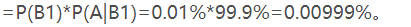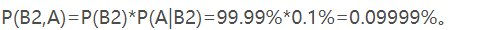然后我们想求得是检查为阳性的情况下，患有贝叶死的概率，也即是 P(B1|A)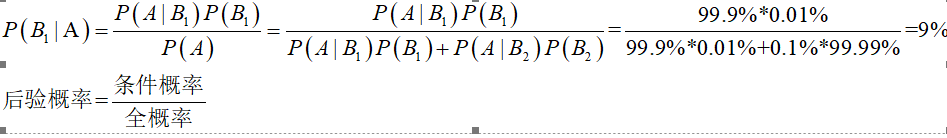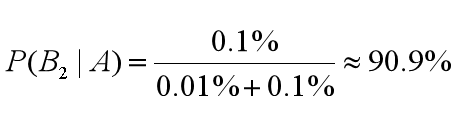朴素贝叶斯
朴素贝叶斯，它是一种简单但极为强大的预测建模算法。之所以称为朴素贝叶斯，**是因为它假设每个输入变量是独立的。**这个假设很硬，现实生活中根本不满足，但是这项技术对于绝大部分的复杂问题仍然非常有效。
朴素贝叶斯模型由两种类型的概率组成： 1、每个类别的概率P(Cj)； 2、每个属性的条件概率P(Ai|Cj)。

我们回归到贝叶死的案例中来，类型概率是患病，不患病；条件概率是：患病的条件下，被检查出阳性的概率，不患病的条件下，检查出阳性的概率（误诊的概率）。要求的被检查出阳性，那么患病的概率（贝叶斯是求后验概率–知道结果，推测原因的概率，“求什么什么是类别，其它的就是属性条件”！）

为了训练朴素贝叶斯模型，我们需要先给出训练数据，以及这些数据对应的分类。那么上面这两个概率，也就是类别概率和条件概率。他们都可以从给出的训练数据中计算出来。一旦计算出来，概率模型就可以使用贝叶斯原理对新数据进行预测。
贝叶斯原理、贝叶斯分类和朴素贝叶斯这三者之间是有区别的 贝叶斯原理是最大的概念，它解决了概率论中“逆向概率”的问题，在这个理论基础上，人们设计出了贝叶斯分类器，朴素贝叶斯分类是贝叶斯分类器中的一种，也是最简单，最常用的分类器。朴素贝叶斯之所以朴素是因为它假设属性是相互独立的，因此对实际情况有所约束，**如果属性之间存在关联，分类准确率会降低。**不过好在对于大部分情况下，朴素贝叶斯的分类效果都不错。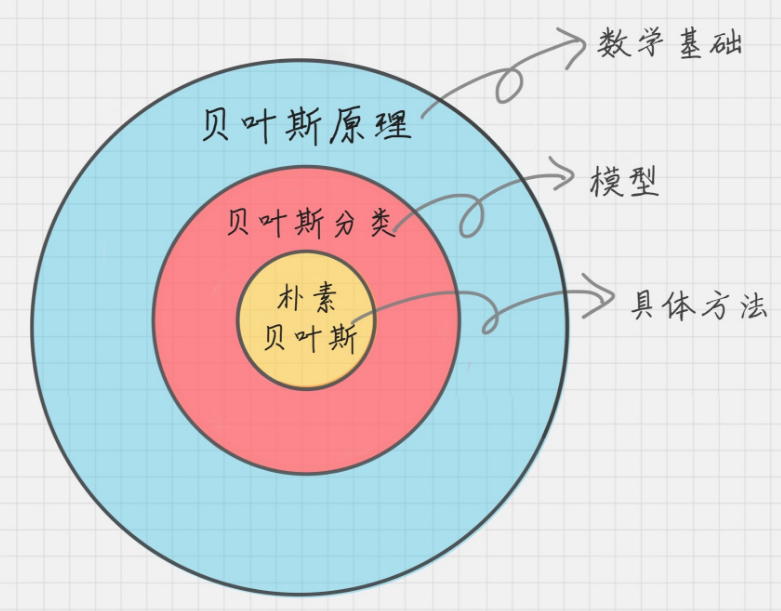离散数据案例
我以下面的数据为例，这些是根据你之前的经验所获得的数据。然后给你一个新的数据：身高“高”、体重“中”，鞋码“中”，请问这个人是男还是女？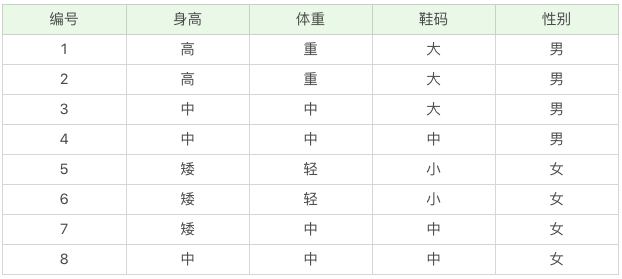男女就是类型，男C1，女C2; 属性条件：身高A1，体重A2，鞋码A3 那么我们想求在 A1、A2、A3 属性下，Cj 的概率，用条件概率表示就是 P(Cj|A1A2A3)。根据上面讲的贝叶斯的公式，我们可以得出：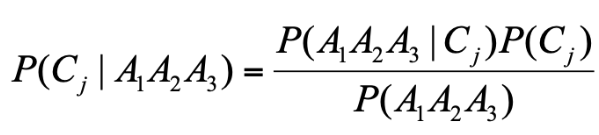因为一共有 2 个类别，所以我们只需要求得 P(C1|A1A2A3) 和P(C2|A1A2A3) 的概率即可，然后比较下哪个分类的可能性大，就是哪个分类结果。 等价于求 P(A1A2A3|Cj)P(Cj) 最大值
我们假定 Ai 之间是相互独立的，那么：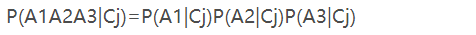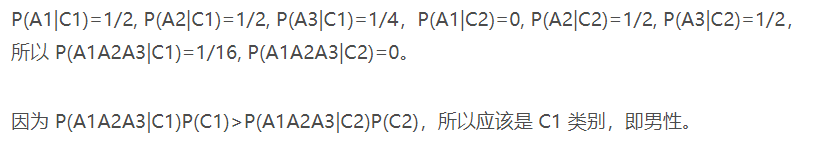连续数据案例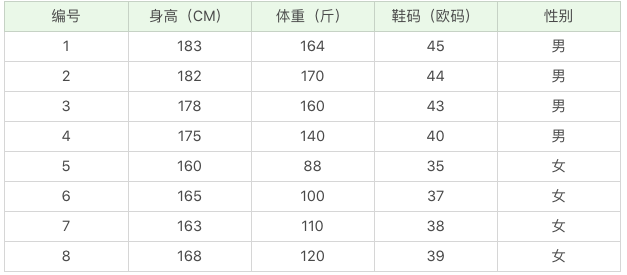那么如果给你一个新的数据，身高 180、体重 120，鞋码 41，请问该人是男是女呢？ 公式还是上面的公式，这里的困难在于，由于身高、体重、鞋码都是连续变量，不能采用离散变量的方法计算概率。而且由于样本太少，所以也无法分成区间计算。怎么办呢？
这时，可以假设男性和女性的身高、体重、鞋码都是正态分布，通过样本计算出均值和方差，也就是得到正态分布的密度函数。 有了密度函数，就可以把值代入，算出某一点的密度函数的值。
比如，男性的身高是均值 179.5、标准差为 3.697 的正态分布。（我们选择不同条件下的样本，得出的均值，标准差就是条件下的概率分布了。这点稍后计算中体现）
所以男性的身高为 180 的概率为 0.1069。怎么计算得出的呢? –excel
NORMDIST(x,mean,standard_dev,cumulative) 函数，一共有 4 个参数：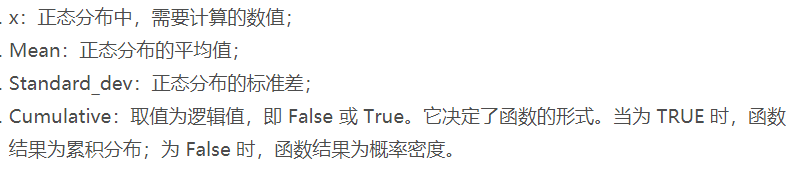这里我们使用的是 NORMDIST(180,179.5,3.697,0)=0.1069。 同理我们可以计算得出男性体重为 120 的概率为 0.000382324， 男性鞋码为 41 号的概率为 0.120304111。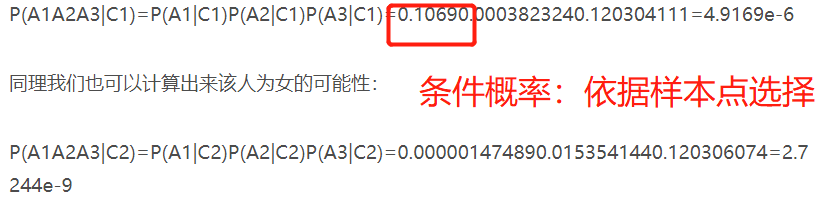很明显这组数据分类为男的概率大于分类为女的概率。
总结：
朴素贝叶斯分类常用于文本分类，尤其是对于英文等语言来说，分类效果很好。它常用于垃圾文本过滤、情感预测、推荐系统等。
第一阶段：准备阶段 在这个阶段我们需要确定特征属性，比如上面案例中的“身高”、“体重”、“鞋码”等，同时明确预测值是什么。并对每个特征属性进行适当划分，然后由人工对一部分数据进行分类，形成训练样本。

这一阶段是整个朴素贝叶斯分类中唯一需要人工完成的阶段，其质量对整个过程将有重要影响，分类器的质量很大程度上由特征属性、特征属性划分及训练样本质量决定。

第二阶段：训练阶段
这个阶段就是生成分类器，主要工作是计算每个类别在训练样本中的出现频率及每个特征属性划分对每个类别的条件概率。

输入是特征属性和训练样本，输出是分类器。

第三阶段：应用阶段
这个阶段是使用分类器对新数据进行分类。

输入是分类器和新数据，输出是新数据的分类结果。

可以考虑：自编程实现第一阶段，第二阶段！
思考题：
如果你的女朋友，在你的手机里发现了和别的女人的暧昧短信，于是她开始思考了 3 个概率问题，你来判断下下面的 3 个概率分别属于哪种概率：
1、你在没有任何情况下，出轨的概率；（先验概率） 2、在你的手机里发现了暧昧短信，认为你出轨的概率。（条件概率） 3、如果你出轨了，那么你的手机里有暧昧短信的概率；（后验概率）
对应到贝叶斯案例 1、假设有一种病叫做“贝叶死”，它的发病率是万分之一， 2、现有一种测试可以检验一个人是否得病的准确率是 99.9%，它的误报率是 0.1% 3、那么现在的问题是，如果一个人被查出来患有“叶贝死”，实际上患有的可能性有多大？
tips：知道客观事实，诊断出病/认为你出轨（主观判断）这是条件概率–知道原因，推测结果的概率。 另一种局面：认为你出轨/诊断出病，推测原因（客观事实）出现的概率这是后验概率（知道结果推测原因发生的概率）
贝叶斯算法的优缺点
优点：     （1）朴素贝叶斯模型发源于古典数学理论，有稳定的分类效率。     （2）对小规模的数据表现很好，能个处理多分类任务，适合增量式训练，尤其是数据量超出内存时，我们可以一批批的去增量训练。     （3）对缺失数据不太敏感，算法也比较简单，常用于文本分类。   缺点：     （1）理论上，朴素贝叶斯模型与其他分类方法相比具有最小的误差率。但是实际上并非总是如此，这是因为朴素贝叶斯模型给定输出类别的情况下,假设属性之间相互独立，这个假设在实际应用中往往是不成立的，在属性个数比较多或者属性之间相关性较大时，分类效果不好。而在属性相关性较小时，朴素贝叶斯性能最为良好。对于这一点，有半朴素贝叶斯之类的算法通过考虑部分关联性适度改进。     （2）需要知道先验概率，且先验概率很多时候取决于假设，假设的模型可以有很多种，因此在某些时候会由于假设的先验模型的原因导致预测效果不佳。     （3）由于我们是通过先验和数据来决定后验的概率从而决定分类，所以分类决策存在一定的错误率。     （4）对输入数据的表达形式很敏感。
参考文献
数据分析实战45讲 统计学习方法（李航） 理论推导看这里：https://www.jianshu.com/p/b6cadf53b8b8
展开全文• 神经网络结构以及前向传播过程 损失函数和代价函数 ...Hello，对于神经网络的原理，我入门了好多次，每次都觉得懂了，但是其实内部原理并没有理解透彻。经过不懈努力，终于茅塞顿开，遂总结此文。 本


文章目录
0. 什么是人工神经网络？1. 神经网络初探1.1 神经元模型1.2 神经元激活函数1.3 神经网络结构
2. 损失函数和代价函数3. 反向传播3.1 矩阵补充知识**3.1.1 求梯度矩阵****3.1.2 海塞矩阵****3.1.3 总结**
3.3 梯度下降法原理3.4 反向传播原理（四个基础等式）3.5 反向传播总结**3.5.1 单样本输入公式表****3.5.2 多样本输入公式表****3.5.3 关于超参数**

4. 是不是猫？4.1 辅助函数4.2 前向传播过程4.3 反向传播过程4.4 测试结果
5. 本文小结

订正与答疑：1. 具体解释一下公式1里面的“堆砌”是什么意思？2. 公式2写成矩阵形式为什么系数矩阵会有转置？自己没搞懂。3. 公式3能具体讲一下矩阵形式是怎么来的吗？4. 为什么会损失函数不用最小二乘法？5. 能不能顺便介绍一下Python环境的管理？6. 为什么w的初始化使用随机初始化，而b参数的初始化全部初始化为0？

本文着重讲述经典BP神经网络的数学推导过程，并辅助一个小例子。本文不会介绍机器学习库(比如sklearn, TensorFlow等)的使用。 欲了解卷积神经网络的内容，请参见我的另一篇博客一文搞定卷积神经网络——从原理到应用。

本文难免会有叙述不合理的地方，希望读者可以在评论区反馈。我会及时吸纳大家的意见，并在之后的chat里进行说明。
本文参考了一些资料，在此一并列出。
http://neuralnetworksanddeeplearning.com/chap2.htmlhttps://www.deeplearning.ai/ coursera对应课程视频讲义coursera华盛顿大学机器学习专项周志华《机器学习》李航《统计学习方法》张明淳《工程矩阵理论》

0. 什么是人工神经网络？
首先给出一个经典的定义：“神经网络是由具有适应性的简单单元组成的广泛并行互连网络，它的组织能够模拟生物神经系统对真实世界物体所作出的交互反应”[Kohonen, 1988]。
这种说法虽然很经典，但是对于初学者并不是很友好。比如我在刚开始学习的时候就把人工神经网络想象地很高端，以至于很长一段时间都不能理解为什么神经网络能够起作用。类比最小二乘法线性回归问题，在求解数据拟合直线的时候，我们是采用某种方法让预测值和实际值的“偏差”尽可能小。同理，BP神经网络也做了类似的事情——即通过让“偏差”尽可能小，使得神经网络模型尽可能好地拟合数据集。
1. 神经网络初探
1.1 神经元模型
神经元模型是模拟生物神经元结构而被设计出来的。典型的神经元结构如下图1所示：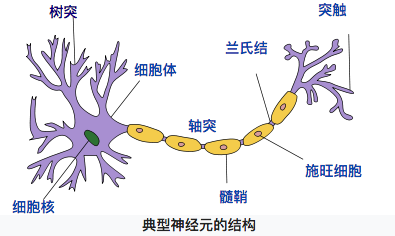【图1 典型神经元结构 （图片来自维基百科）】
神经元大致可以分为树突、突触、细胞体和轴突。树突为神经元的输入通道，其功能是将其它神经元的动作电位传递至细胞体。其它神经元的动作电位借由位于树突分支上的多个突触传递至树突上。神经细胞可以视为有两种状态的机器，激活时为“是”，不激活时为“否”。神经细胞的状态取决于从其他神经细胞接收到的信号量，以及突触的性质（抑制或加强）。当信号量超过某个阈值时，细胞体就会被激活，产生电脉冲。电脉冲沿着轴突并通过突触传递到其它神经元。（内容来自维基百科“感知机”）
同理，我们的神经元模型就是为了模拟上述过程，典型的神经元模型如下：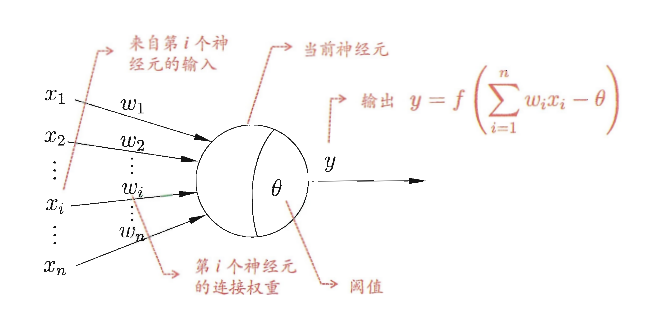【图2 典型神经元模型结构 （摘自周志华老师《机器学习》第97页）】
这个模型中，每个神经元都接受来自其它神经元的输入信号，每个信号都通过一个带有权重的连接传递，神经元把这些信号加起来得到一个总输入值，然后将总输入值与神经元的阈值进行对比（模拟阈值电位），然后通过一个“激活函数”处理得到最终的输出（模拟细胞的激活），这个输出又会作为之后神经元的输入一层一层传递下去。
1.2 神经元激活函数
本文主要介绍2种激活函数，分别是

s

i

g

m

o

i

d

sigmoid

和

r

e

l

u

relu

函数，函数公式如下：

s

i

g

m

o

i

d

(

z

)

=

1

1

+

e

−

z

sigmoid(z)=\frac{1}{1+e^{-z}}

r

e

l

u

(

z

)

=

{

z

z

&gt;

0

0

z

≤

0

relu(z)= \left\{ \begin{array}{rcl} z &amp; z&gt;0\\ 0&amp;z\leq0\end{array} \right.

做函数图如下：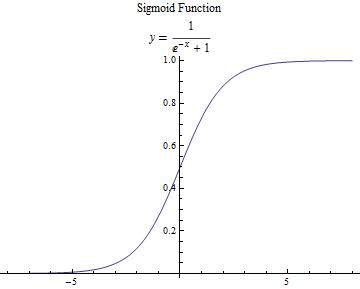s

i

g

m

o

i

d

(

z

)

sigmoid(z)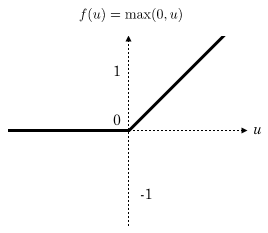r

e

l

u

(

z

)

relu(z)

【图3 激活函数】

补充说明 【补充说明的内容建议在看完后文的反向传播部分之后再回来阅读，我只是为了文章结构的统一把这部分内容添加在了这里】
引入激活函数的目的是在模型中引入非线性。如果没有激活函数，那么无论你的神经网络有多少层，最终都是一个线性映射，单纯的线性映射无法解决线性不可分问题。引入非线性可以让模型解决线性不可分问题。
一般来说，在神经网络的中间层更加建议使用

r

e

l

u

relu

函数，两个原因：

r

e

l

u

relu

函数计算简单，可以加快模型速度；由于反向传播过程中需要计算偏导数，通过求导可以得到

s

i

g

m

o

i

d

sigmoid

函数导数的最大值为0.25，如果使用

s

i

g

m

o

i

d

sigmoid

函数的话，每一层的反向传播都会使梯度最少变为原来的四分之一，当层数比较多的时候可能会造成梯度消失，从而模型无法收敛。

1.3 神经网络结构
我们使用如下神经网络结构来进行介绍，第0层是输入层（3个神经元）， 第1层是隐含层（2个神经元），第2层是输出层：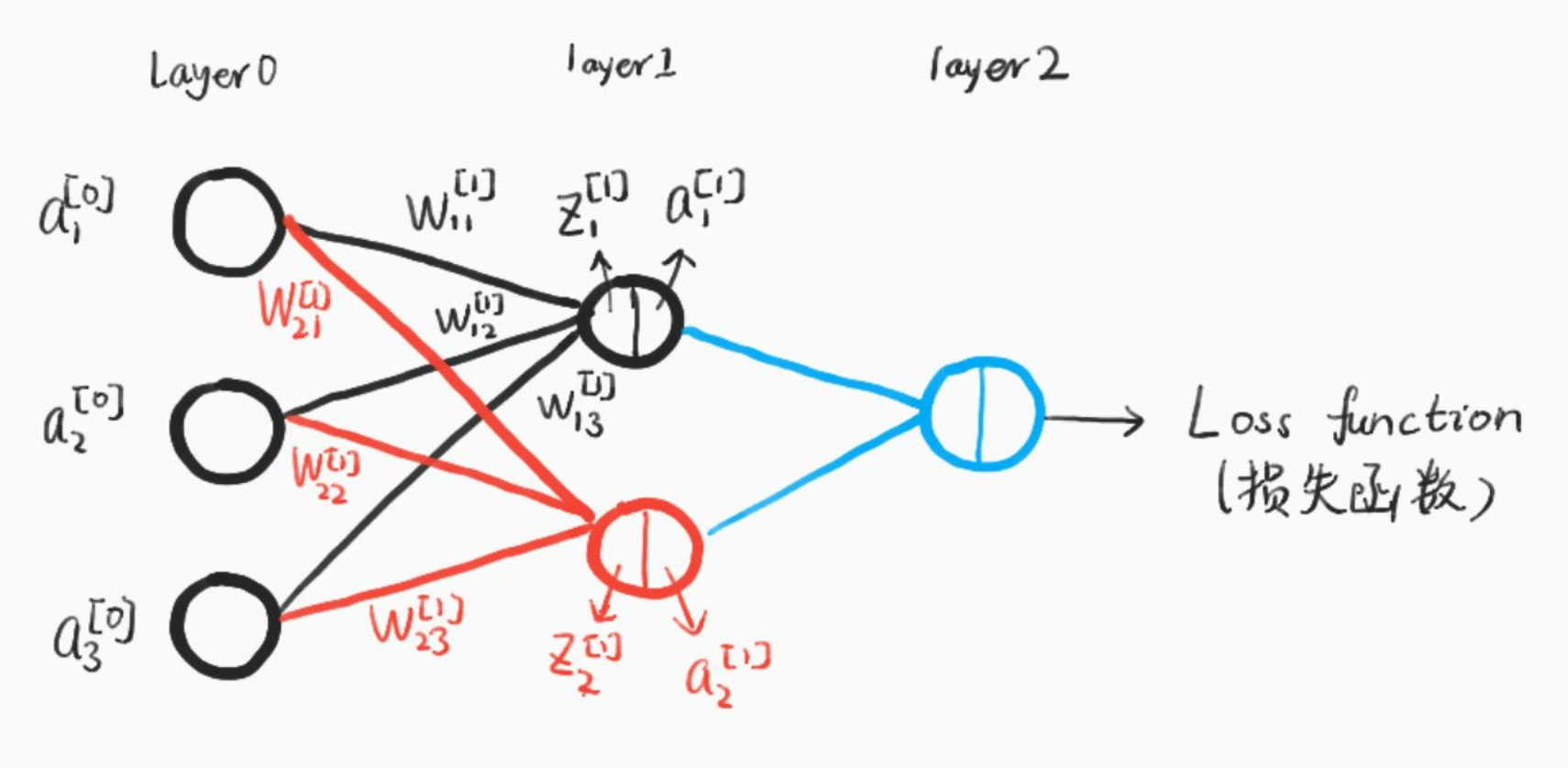【图4 神经网络结构（手绘）】
我们使用以下符号约定，

w

j

k

[

l

]

w_{jk}^{[l]}

表示从网络第

(

l

−

1

)

t

h

(l-1)^{th}

中

k

t

h

k^{th}

个神经元指向第

l

t

h

l^{th}

中第

j

t

h

j^{th}

个神经元的连接权重，比如上图中

w

21

[

1

]

w^{}_{21}

即从第0层第1个神经元指向第1层第2个神经元的权重。同理，我们使用

b

j

[

l

]

b^{[l]}_j

来表示第

l

t

h

l^{th}

层中第

j

t

h

j^{th}

神经元的偏差，用

z

j

[

l

]

z^{[l]}_j

来表示第

l

t

h

l^{th}

层中第

j

t

h

j^{th}

神经元的线性结果,用

a

j

[

l

]

a^{[l]}_j

来表示第

l

t

h

l^{th}

层中第

j

t

h

j^{th}

神经元的激活函数输出。
激活函数使用符号

σ

\sigma

表示，因此，第

l

t

h

l^{th}

层中第

j

t

h

j^{th}

神经元的激活为：

a

j

[

l

]

=

σ

(

∑

k

w

j

k

[

l

]

a

k

[

l

−

1

]

+

b

j

[

l

]

)

a^{[l]}_j=\sigma(\sum_kw^{[l]}_{jk}a^{[l-1]}_k+b^{[l]}_j)

现在，我们使用矩阵形式重写这个公式：
定义

w

[

l

]

w^{[l]}

表示权重矩阵，它的每一个元素表示一个权重，即每一行都是连接第

l

l

层的权重，用上图举个例子就是：

w

[

1

]

=

[

w

11

[

1

]

w

12

[

1

]

w

13

[

1

]

w

21

[

1

]

w

22

[

1

]

w

23

[

1

]

]

w^{}=\left[ \begin{array}{cc} w_{11}^{} &amp; w_{12}^{} &amp; w_{13}^{} \\ w_{21}^{}&amp; w_{22}^{} &amp; w_{23}^{}\end{array}\right]

同理，

b

[

1

]

=

[

b

1

[

1

]

b

2

[

1

]

]

b^{}=\left[ \begin{array}{cc}b^{}_1 \\ b^{}_2 \end{array}\right]

z

[

1

]

=

[

w

11

[

1

]

w

12

[

1

]

w

13

[

1

]

w

21

[

1

]

w

22

[

1

]

w

23

[

1

]

]

⋅

[

a

1

[

0

]

a

2

[

0

]

a

3

[

0

]

]

+

[

b

1

[

1

]

b

2

[

1

]

]

=

[

w

11

[

1

]

a

1

[

0

]

+

w

12

[

1

]

a

2

[

0

]

+

w

13

[

1

]

a

3

[

0

]

+

b

1

[

1

]

w

21

[

1

]

a

1

[

0

]

+

w

22

[

1

]

a

2

[

0

]

+

w

23

[

1

]

a

3

[

0

]

+

b

2

[

1

]

]

z^{}=\left[ \begin{array}{cc} w_{11}^{} &amp; w_{12}^{} &amp; w_{13}^{} \\ w_{21}^{}&amp; w_{22}^{} &amp; w_{23}^{}\end{array}\right]\cdot \left[ \begin{array}{cc} a^{}_1 \\ a^{}_2 \\ a^{}_3 \end{array}\right] +\left[ \begin{array}{cc}b^{}_1 \\ b^{}_2 \end{array}\right]=\left[ \begin{array}{cc} w_{11}^{}a^{}_1+w_{12}^{}a^{}_2+w_{13}^{}a^{}_3+b^{}_1 \\ w^{}_{21}a^{}_1+w_{22}^{}a^{}_2+w_{23}^{}a^{}_3+b^{}_2\end{array}\right]

更一般地，我们可以把前向传播过程表示：

a

[

l

]

=

σ

(

w

[

l

]

a

[

l

−

1

]

+

b

[

l

]

)

a^{[l]}=\sigma(w^{[l]}a^{[l-1]}+b^{[l]})

到这里，我们已经讲完了前向传播的过程，值得注意的是，这里我们只有一个输入样本，对于多个样本同时输入的情况是一样的，只不过我们的输入向量不再是一列，而是m列，每一个都表示一个输入样本。
多样本输入情况下的表示为：

Z

[

l

]

=

w

[

l

]

⋅

A

[

l

−

1

]

+

b

[

l

]

Z^{[l]}=w^{[l]}\cdot A^{[l-1]}+b^{[l]}

A

[

l

]

=

σ

(

Z

[

l

]

)

A^{[l]}=\sigma(Z^{[l]})

其中，此时

A

[

l

−

1

]

=

[

∣

∣

…

∣

a

[

l

−

1

]

(

1

)

a

[

l

−

1

]

(

2

)

…

a

[

l

−

1

]

(

m

)

∣

∣

…

∣

]

A^{[l-1]}=\left[ \begin{array}{cc} |&amp;|&amp;\ldots&amp;| \\a^{[l-1](1)}&amp;a^{[l-1](2)}&amp;\ldots&amp;a^{[l-1](m)} \\ |&amp;|&amp;\ldots&amp;|\end{array}\right]

每一列都表示一个样本，从样本1到m

w

[

l

]

w^{[l]}

的含义和原来完全一样，

Z

[

l

]

Z^{[l]}

也会变成m列，每一列表示一个样本的计算结果。

之后我们的叙述都是先讨论单个样本的情况，再扩展到多个样本同时计算。

2. 损失函数和代价函数
说实话，**损失函数（Loss Function）和代价函数（Cost Function）**并没有一个公认的区分标准，很多论文和教材似乎把二者当成了差不多的东西。
为了后面描述的方便，我们把二者稍微做一下区分（这里的区分仅仅对本文适用，对于其它的文章或教程需要根据上下文自行判断含义）：

损失函数主要指的是对于单个样本的损失或误差；代价函数表示多样本同时输入模型的时候总体的误差——每个样本误差的和然后取平均值。

举个例子，如果我们把单个样本的损失函数定义为：

L

(

a

,

y

)

=

−

[

y

⋅

l

o

g

(

a

)

+

(

1

−

y

)

⋅

l

o

g

(

1

−

a

)

]

L(a,y)=-[y \cdot log(a)+(1-y)\cdot log(1-a)]

那么对于m个样本，代价函数则是：

C

=

−

1

m

∑

i

=

0

m

(

y

(

i

)

⋅

l

o

g

(

a

(

i

)

)

+

(

1

−

y

(

i

)

)

⋅

l

o

g

(

1

−

a

(

i

)

)

)

C=-\frac{1}{m}\sum_{i=0}^m(y^{(i)}\cdot log(a^{(i)})+(1-y^{(i)})\cdot log(1-a^{(i)}))

3. 反向传播
反向传播的基本思想就是通过计算输出层与期望值之间的误差来调整网络参数，从而使得误差变小。
反向传播的思想很简单，然而人们认识到它的重要作用却经过了很长的时间。后向传播算法产生于1970年，但它的重要性一直到David Rumelhart，Geoffrey Hinton和Ronald Williams于1986年合著的论文发表才被重视。
事实上，人工神经网络的强大力量几乎就是建立在反向传播算法基础之上的。反向传播基于四个基础等式，数学是优美的，仅仅四个等式就可以概括神经网络的反向传播过程，然而理解这种优美可能需要付出一些脑力。事实上，反向传播如此之难，以至于相当一部分初学者很难进行独立推导。所以如果读者是初学者，希望读者可以耐心地研读本节。对于初学者，我觉得拿出1-3个小时来学习本小节是比较合适的，当然，对于熟练掌握反向传播原理的读者，你可以在十几分钟甚至几分钟之内快速浏览本节的内容。
3.1 矩阵补充知识
对于大部分理工科的研究生，以及学习过矩阵论或者工程矩阵理论相关课程的读者来说，可以跳过本节。
本节主要面向只学习过本科线性代数课程或者已经忘记矩阵论有关知识的读者。
总之，具备了本科线性代数知识的读者阅读这一小节应该不会有太大问题。本节主要在线性代数的基础上做一些扩展。（不排除少数本科线性代数课程也涉及到这些内容，如果感觉讲的简单的话，勿喷）
3.1.1 求梯度矩阵
假设函数

f

:

R

m

×

n

→

R

f:R^{m\times n}\rightarrow R

可以把输入矩阵（shape:

m

×

n

m\times n

）映射为一个实数。那么，函数

f

f

的梯度定义为：

∇

A

f

(

A

)

=

[

∂

f

(

A

)

∂

A

11

∂

f

(

A

)

∂

A

12

…

∂

f

(

A

)

∂

A

1

n

∂

f

(

A

)

∂

A

21

∂

f

(

A

)

∂

A

22

…

∂

f

(

A

)

∂

A

2

n

⋮

⋮

⋱

⋮

∂

f

(

A

)

∂

A

m

1

∂

f

(

A

)

∂

A

m

2

…

∂

f

(

A

)

∂

A

m

n

]

\nabla_Af(A)=\left[ \begin{array}{cc} \frac{\partial f(A)}{\partial A_{11}}&amp;\frac{\partial f(A)}{\partial A_{12}}&amp;\ldots&amp;\frac{\partial f(A)}{\partial A_{1n}} \\ \frac{\partial f(A)}{\partial A_{21}}&amp;\frac{\partial f(A)}{\partial A_{22}}&amp;\ldots&amp;\frac{\partial f(A)}{\partial A_{2n}} \\\vdots &amp;\vdots &amp;\ddots&amp;\vdots\\ \frac{\partial f(A)}{\partial A_{m1}}&amp;\frac{\partial f(A)}{\partial A_{m2}}&amp;\ldots&amp;\frac{\partial f(A)}{\partial A_{mn}}\end{array}\right]

即

(

∇

A

f

(

A

)

)

i

j

=

∂

f

(

A

)

∂

A

i

j

(\nabla_Af(A))_{ij}=\frac{\partial f(A)}{\partial A_{ij}}

同理，一个输入是向量（向量一般指列向量，本文在没有特殊声明的情况下默认指的是列向量）的函数

f

:

R

n

×

1

→

R

f:R^{n\times 1}\rightarrow R

，则有：

∇

x

f

(

x

)

=

[

∂

f

(

x

)

∂

x

1

∂

f

(

x

)

∂

x

2

⋮

∂

f

(

x

)

∂

x

n

]

\nabla_xf(x)=\left[ \begin{array}{cc}\frac{\partial f(x)}{\partial x_1}\\ \frac{\partial f(x)}{\partial x_2}\\ \vdots \\ \frac{\partial f(x)}{\partial x_n} \end{array}\right]

注意：这里涉及到的梯度求解的前提是函数

f

f

返回的是一个实数，如果函数返回的是一个矩阵或者向量，那么我们是没有办法求梯度的。比如，对函数

f

(

A

)

=

∑

i

=

0

m

∑

j

=

0

n

A

i

j

2

f(A)=\sum_{i=0}^m\sum_{j=0}^nA_{ij}^2

，由于返回一个实数,我们可以求解梯度矩阵。如果

f

(

x

)

=

A

x

(

A

∈

R

m

×

n

,

x

∈

R

n

×

1

)

f(x)=Ax (A\in R^{m\times n}, x\in R^{n\times 1})

，由于函数返回一个

m

m

行1列的向量，因此不能对

f

f

求梯度矩阵。

根据定义，很容易得到以下性质：

∇

x

(

f

(

x

)

+

g

(

x

)

)

=

∇

x

f

(

x

)

+

∇

x

g

(

x

)

\nabla_x(f(x)+g(x))=\nabla_xf(x)+\nabla_xg(x)

∇

(

t

f

(

x

)

)

=

t

∇

f

(

x

)

,

t

∈

R

\nabla(tf(x))=t\nabla f(x), t\in R

有了上述知识，我们来举个例子：

定义函数

f

:

R

m

→

R

,

f

(

z

)

=

z

T

z

f:R^m\rightarrow R, f(z)=z^Tz

,那么很容易得到

∇

z

f

(

z

)

=

2

z

\nabla_zf(z)=2z

，具体请读者自己证明。

3.1.2 海塞矩阵
定义一个输入为

n

n

维向量，输出为实数的函数

f

:

R

n

→

R

f:R^n\rightarrow R

，那么海塞矩阵（Hessian Matrix）定义为多元函数

f

f

的二阶偏导数构成的方阵：

∇

x

2

f

(

x

)

=

[

∂

2

f

(

x

)

∂

x

1

2

∂

2

f

(

x

)

∂

x

1

∂

x

2

…

∂

2

f

(

x

)

∂

x

1

∂

x

n

∂

2

f

(

x

)

∂

x

2

∂

x

1

∂

2

f

(

x

)

∂

x

2

2

…

∂

2

f

(

x

)

∂

x

2

∂

x

n

⋮

⋮

⋱

⋮

∂

2

f

(

x

)

∂

x

n

∂

x

1

∂

2

f

(

x

)

∂

x

n

∂

x

2

…

∂

2

f

(

x

)

∂

x

n

2

]

\nabla^2_xf(x)=\left[ \begin{array}{cc} \frac{\partial^2f(x)}{\partial x_1^2}&amp;\frac{\partial^2f(x)}{\partial x_1\partial x_2}&amp;\ldots &amp;\frac{\partial^2f(x)}{\partial x_1\partial x_n}\\ \frac{\partial^2f(x)}{\partial x_2\partial x_1}&amp;\frac{\partial^2f(x)}{\partial x_2^2}&amp;\ldots&amp;\frac{\partial^2f(x)}{\partial x_2\partial x_n}\\ \vdots&amp;\vdots&amp;\ddots&amp;\vdots\\\frac{\partial^2f(x)}{\partial x_n\partial x_1}&amp;\frac{\partial^2f(x)}{\partial x_n\partial x_2}&amp;\ldots&amp;\frac{\partial^2f(x)}{\partial x_n^2}\end{array}\right]

由上式可以看出，海塞矩阵总是对称阵。

注意：很多人把海塞矩阵看成

∇

x

f

(

x

)

\nabla _xf(x)

的导数，这是不对的。只能说，海塞矩阵的每个元素都是函数

f

f

二阶偏导数。那么，有什么区别呢？
首先，来看正确的解释。**海塞矩阵的每个元素是函数

f

f

的二阶偏导数。**拿

∂

2

f

(

x

)

∂

x

1

∂

x

2

\frac{\partial^2f(x)}{\partial x_1\partial x_2}

举个例子，函数

f

f

对

x

1

x_1

求偏导得到的是一个实数，比如

∂

2

f

(

x

)

∂

x

1

=

x

2

3

x

1

\frac{\partial^2f(x)}{\partial x_1}=x_2^3x_1

，因此继续求偏导是有意义的,继续对

x

2

x_2

求偏导可以得到

3

x

1

x

2

2

3x_1x_2^2

。
然后,来看一下错误的理解。把海塞矩阵看成

∇

x

f

(

x

)

\nabla _xf(x)

的导数，也就是说错误地以为

∇

x

2

f

(

x

)

=

∇

x

(

∇

x

f

(

x

)

)

\nabla^2_xf(x)=\nabla_x(\nabla_xf(x))

，要知道，

∇

x

f

(

x

)

\nabla_xf(x)

是一个向量，而在上一小节我们已经重点强调过，在我们的定义里对向量求偏导是没有定义的。
但是

∇

x

∂

f

(

x

)

∂

x

i

\nabla_x\frac{\partial f(x)}{\partial x_i}

是有意义的，因为

∂

f

(

x

)

∂

x

i

\frac{\partial f(x)}{\partial x_i}

是一个实数，具体地：

∇

x

∂

f

(

x

)

∂

x

i

=

[

∂

2

f

(

x

)

∂

x

i

∂

x

1

∂

2

f

(

x

)

∂

x

i

∂

x

2

⋮

∂

2

f

(

x

)

∂

x

i

∂

x

n

]

\nabla_x\frac{\partial f(x)}{\partial x_i}=\left[ \begin{array}{cc} \frac{\partial^2f(x)}{\partial x_i\partial x_1}\\\frac{\partial^2f(x)}{\partial x_i\partial x_2}\\\vdots\\\frac{\partial^2f(x)}{\partial x_i\partial x_n}\end{array}\right]

即海塞矩阵的第i行（或列）。
希望读者可以好好区分。

3.1.3 总结
根据3.1.1和3.1.2小节的内容很容易得到以下等式：

b

∈

R

n

,

x

∈

R

n

,

A

∈

R

n

×

n

并

且

A

是

对

称

矩

阵

b\in R^{n}, x\in R^n, A\in R^{n\times n}并且A 是对称矩阵

b

,

x

b,x

均为列向量   那么，

∇

x

b

T

x

=

b

\nabla_xb^Tx=b

∇

x

x

T

A

x

=

2

A

x

(

A

是

对

称

阵

)

\nabla_xx^TAx=2Ax(A是对称阵)

∇

x

2

x

T

A

x

=

2

A

(

A

是

对

称

阵

)

\nabla^2_xx^TAx=2A(A是对称阵)

这些公式可以根据前述定义自行推导，有兴趣的读者可以自己推导一下。 ####3.2 矩阵乘积和对应元素相乘   在下一节讲解反向传播原理的时候，尤其是把公式以矩阵形式表示的时候，需要大家时刻区分什么时候需要矩阵相乘，什么时候需要对应元素相乘。

比如对于矩阵

A

=

[

1

2

3

4

]

，

矩

阵

B

=

[

−

1

−

2

−

3

−

4

]

A=\left[ \begin{array}{cc} 1&amp;2\\3&amp;4\end{array}\right]，矩阵B=\left[ \begin{array}{cc} -1&amp;-2\\-3&amp;-4\end{array}\right]

矩阵相乘

A

B

=

[

1

×

−

1

+

2

×

−

3

1

×

−

2

+

2

×

−

4

3

×

−

1

+

4

×

−

3

3

×

−

2

+

4

×

−

4

]

=

[

−

7

−

10

−

15

−

22

]

AB=\left[\begin{array}{cc}1\times -1+2\times -3&amp;1\times -2+2\times -4\\3\times -1+4\times -3&amp;3\times -2+4\times -4\end{array}\right]=\left[\begin{array}{cc}-7&amp;-10\\-15&amp;-22\end{array}\right]

对应元素相乘使用符号

⊙

\odot

表示：

A

⊙

B

=

[

1

×

−

1

2

×

−

2

3

×

−

3

4

×

−

4

]

=

[

−

1

−

4

−

9

−

16

]

A\odot B=\left[\begin{array}{cc}1\times -1&amp;2\times -2 \\ 3\times -3&amp;4\times -4\end{array}\right]=\left[\begin{array}{cc}-1&amp;-4 \\ -9&amp;-16\end{array}\right]

3.3 梯度下降法原理
通过之前的介绍，相信大家都可以自己求解梯度矩阵（向量）了。
那么梯度矩阵（向量）求出来的意义是什么？从几何意义讲，梯度矩阵代表了函数增加最快的方向，因此，沿着与之相反的方向就可以更快找到最小值。如图5所示：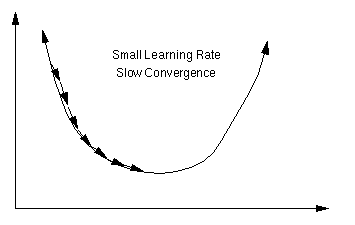【图5 梯度下降法 图片来自百度】   反向传播的过程就是利用梯度下降法原理，慢慢的找到代价函数的最小值，从而得到最终的模型参数。梯度下降法在反向传播中的具体应用见下一小节。
3.4 反向传播原理（四个基础等式）
反向传播能够知道如何更改网络中的权重

w

w

和偏差

b

b

来改变代价函数值。最终这意味着它能够计算偏导数

∂

L

(

a

[

l

]

,

y

)

∂

w

j

k

[

l

]

\frac{\partial L(a^{[l]},y)} {\partial w^{[l]}_{jk}}

和

∂

L

(

a

[

l

]

,

y

)

∂

b

j

[

l

]

\frac{\partial L(a^{[l]},y)}{\partial b^{[l]}_j}

为了计算这些偏导数，我们首先引入一个中间变量

δ

j

[

l

]

\delta^{[l]}_j

，我们把它叫做网络中第

l

t

h

l^{th}

层第

j

t

h

j^{th}

个神经元的误差。后向传播能够计算出误差

δ

j

[

l

]

\delta^{[l]}_j

，然后再将其对应回

∂

L

(

a

[

l

]

,

y

)

∂

w

j

k

[

l

]

\frac{\partial L(a^{[l]},y)}{\partial w^{[l]}_{jk}}

和

∂

L

(

a

[

l

]

,

y

)

∂

b

j

[

l

]

\frac{\partial L(a^{[l]},y)}{\partial b^{[l]}_j}

。
那么，如何定义每一层的误差呢？如果为第

l

l

层第

j

j

个神经元添加一个扰动

Δ

z

j

[

l

]

\Delta z^{[l]}_j

，使得损失函数或者代价函数变小，那么这就是一个好的扰动。通过选择

Δ

z

j

[

l

]

\Delta z^{[l]}_j

与

∂

L

(

a

[

l

]

,

y

)

∂

z

j

[

l

]

\frac{\partial L(a^{[l]}, y)}{\partial z^{[l]}_j}

符号相反（梯度下降法原理），就可以每次都添加一个好的扰动最终达到最优。
受此启发，我们定义网络层第

l

l

层中第

j

j

个神经元的误差为

δ

j

[

l

]

\delta^{[l]}_j

:

δ

j

[

l

]

=

∂

L

(

a

[

L

]

,

y

)

∂

z

j

[

l

]

\delta^{[l]}_j=\frac{\partial L(a^{[L], y})}{\partial z^{[l]}_j}

于是，每一层的误差向量可以表示为：

δ

[

l

]

=

[

δ

1

[

l

]

δ

2

[

l

]

⋮

δ

n

[

l

]

]

\delta ^{[l]}=\left[\begin{array}{cc}\delta ^{[l]}_1\\\delta ^{[l]}_2\\ \vdots \\ \delta ^{[l]}_n\end{array} \right]

下面开始正式介绍四个基础等式【确切的说是四组等式】

**注意：**这里我们的输入为单个样本(所以我们在下面的公式中使用的是损失函数而不是代价函数)。多个样本输入的公式会在介绍完单个样本后再介绍。

等式1 ：输出层误差

δ

j

[

L

]

=

∂

L

∂

a

j

[

L

]

σ

′

(

z

j

[

L

]

)

\delta^{[L]}_j=\frac{\partial L}{\partial a^{[L]}_j}\sigma^{&#x27;}(z^{[L]}_j)

其中，

L

L

表示输出层层数。以下用

∂

L

\partial L

表示

∂

L

(

a

[

L

]

,

y

)

\partial L(a^{[L]}, y)

写成矩阵形式是：

δ

[

L

]

=

∇

a

L

⊙

σ

′

(

z

[

L

]

)

\delta^{[L]}=\nabla _aL\odot \sigma^{&#x27;}(z^{[L]})

【注意是对应元素相乘，想想为什么？】

说明
根据本小节开始时的叙述，我们期望找到

∂

L

/

∂

z

j

[

l

]

\partial L \ /\partial z^{[l]}_j

，然后朝着方向相反的方向更新网络参数，并定义误差为：

δ

j

[

L

]

=

∂

L

∂

z

j

[

L

]

\delta^{[L]}_j=\frac{\partial L}{\partial z^{[L]}_j}

根据链式法则，

δ

j

[

L

]

=

∑

k

∂

L

∂

a

k

[

L

]

∂

a

k

[

L

]

∂

z

j

[

L

]

\delta^{[L]}_j = \sum_k \frac{\partial L}{\partial a^{[L]}_k} \frac{\partial a^{[L]}_k}{\partial z^{[L]}_j}

当

k

≠

j

k\neq j

时，

∂

a

k

[

L

]

/

∂

z

j

[

L

]

\partial a^{[L]}_k / \partial z^{[L]}_j

就为零。结果我们可以简化之前的等式为

δ

j

[

L

]

=

∂

L

∂

a

j

[

L

]

∂

a

j

[

L

]

∂

z

j

[

L

]

\delta^{[L]}_j = \frac{\partial L}{\partial a^{[L]}_j} \frac{\partial a^{[L]}_j}{\partial z^{[L]}_j}

重新拿出定义：

a

j

[

L

]

=

σ

(

z

j

[

L

]

)

a^{[L]}_j = \sigma(z^{[L]}_j)

，就可以得到：

δ

j

[

L

]

=

∂

L

∂

a

j

[

L

]

σ

′

(

z

j

[

L

]

)

\delta^{[L]}_j = \frac{\partial L}{\partial a^{[L]}_j} \sigma&#x27;(z^{[L]}_j)

再"堆砌"成向量形式就得到了我们的矩阵表示式（这也是为什么使用矩阵形式表示需要 对应元素相乘 的原因）。

等式2： 隐含层误差

δ

j

[

l

]

=

∑

k

w

k

j

[

l

+

1

]

δ

k

[

l

+

1

]

σ

′

(

z

j

[

l

]

)

\delta^{[l]}_j = \sum_k w^{[l+1]}_{kj} \delta^{[l+1]}_k \sigma&#x27;(z^{[l]}_j)

写成矩阵形式：

δ

[

l

]

=

[

w

[

l

+

1

]

T

δ

[

l

+

1

]

]

⊙

σ

′

(

z

[

l

]

)

\delta^{[l]}=[w^{[l+1]T}\delta^{[l+1]}]\odot \sigma ^{&#x27;}(z^{[l]})

说明：

z

k

[

l

+

1

]

=

∑

j

w

k

j

[

l

+

1

]

a

j

[

l

]

+

b

k

[

l

+

1

]

=

∑

j

w

k

j

[

l

+

1

]

σ

(

z

j

[

l

]

)

+

b

k

[

l

+

1

]

z^{[l+1]}_k=\sum_jw^{[l+1]}_{kj}a^{[l]}_j+b^{[l+1]}_k=\sum_jw^{[l+1]}_{kj}\sigma(z^{[l]}_j)+b^{[l+1]}_k

进行偏导可以获得：

∂

z

k

[

l

+

1

]

∂

z

j

[

l

]

=

w

k

j

[

l

+

1

]

σ

′

(

z

j

[

l

]

)

\frac{\partial z^{[l+1]}_k}{\partial z^{[l]}_j} = w^{[l+1]}_{kj} \sigma&#x27;(z^{[l]}_j)

代入得到：

δ

j

[

l

]

=

∑

k

w

k

j

[

l

+

1

]

δ

k

[

l

+

1

]

σ

′

(

z

j

[

l

]

)

\delta^{[l]}_j = \sum_k w^{[l+1]}_{kj} \delta^{[l+1]}_k \sigma&#x27;(z^{[l]}_j)

等式3：参数变化率

∂

L

∂

b

j

[

l

]

=

δ

j

[

l

]

\frac{\partial L}{\partial b^{[l]}_j}=\delta^{[l]}_j

∂

L

∂

w

j

k

[

l

]

=

a

k

[

l

−

1

]

δ

j

[

l

]

\frac{\partial L}{\partial w^{[l]}_{jk}}=a^{[l-1]}_k\delta^{[l]}_j

写成矩阵形式：

∂

L

∂

b

[

l

]

=

δ

[

l

]

\frac{\partial L}{\partial b^{[l]}}=\delta^{[l]}

∂

L

∂

w

[

l

]

=

δ

[

l

]

a

[

l

−

1

]

T

\frac{\partial L}{\partial w^{[l]}}=\delta^{[l]}a^{[l-1]T}

说明：
根据链式法则推导。   由于

z

j

[

l

]

=

∑

k

w

j

k

[

l

]

a

k

[

l

]

+

b

k

[

l

]

z^{[l]}_j=\sum_kw^{[l]}_{jk}a^{[l]}_k+b^{[l]}_k

对

b

j

[

l

]

b^{[l]}_j

求偏导得到：

∂

L

∂

b

j

[

l

]

=

∂

L

∂

z

j

[

l

]

∂

z

j

[

l

]

b

j

[

l

]

=

δ

j

[

l

]

\frac{\partial L}{\partial b^{[l]}_j}=\frac{\partial L}{\partial z^{[l]}_j}\frac{\partial z^{[l]}_j}{b^{[l]}_j}=\delta^{[l]}_j

对

w

j

k

[

l

]

w^{[l]}_{jk}

求偏导得到：

∂

L

∂

w

j

k

[

l

]

=

∂

L

∂

z

j

[

l

]

∂

z

j

[

l

]

w

j

k

[

l

]

=

a

k

[

l

−

1

]

δ

j

[

l

]

\frac{\partial L}{\partial w^{[l]}_{jk}}=\frac{\partial L}{\partial z^{[l]}_j}\frac{\partial z^{[l]}_j}{w^{[l]}_{jk}}=a^{[l-1]}_k\delta^{[l]}_j

最后再变成矩阵形式就好了。

对矩阵形式来说，需要特别注意维度的匹配。强烈建议读者在自己编写程序之前，先列出这些等式，然后仔细检查维度是否匹配。

很容易看出

∂

L

∂

w

[

l

]

\frac{\partial L}{\partial w^{[l]}}

是一个

d

i

m

(

δ

[

l

]

)

dim(\delta^{[l]})

行

d

i

m

(

a

[

l

−

1

]

)

dim(a^{[l-1]})

列的矩阵，和

w

[

l

]

w^{[l]}

的维度一致；

∂

L

∂

b

[

l

]

\frac{\partial L}{\partial b^{[l]}}

是一个维度为

d

i

m

(

δ

[

l

]

)

dim(\delta^{[l]})

的列向量

等式4：参数更新规则
这应该是这四组公式里最简单的一组了，根据梯度下降法原理，朝着梯度的反方向更新参数：

b

j

[

l

]

←

b

j

[

l

]

−

α

∂

L

∂

b

j

[

l

]

b^{[l]}_j\leftarrow b^{[l]}_j-\alpha \frac{\partial L}{\partial b^{[l]}_j}

w

j

k

[

l

]

←

w

j

k

[

l

]

−

α

∂

L

∂

w

j

k

[

l

]

w^{[l]}_{jk}\leftarrow w^{[l]}_{jk}-\alpha\frac{\partial L}{\partial w^{[l]}_{jk}}

写成矩阵形式：

b

[

l

]

←

b

[

l

]

−

α

∂

L

∂

b

[

l

]

b^{[l]}\leftarrow b^{[l]}-\alpha\frac{\partial L}{\partial b^{[l]}}

w

[

l

]

←

w

[

l

]

−

α

∂

L

∂

w

[

l

]

w^{[l]}\leftarrow w^{[l]}-\alpha\frac{\partial L}{\partial w^{[l]}}

这里的

α

\alpha

指的是学习率。学习率指定了反向传播过程中梯度下降的步长。

3.5 反向传播总结
我们可以得到如下最终公式：
3.5.1 单样本输入公式表
说明公式备注输出层误差

δ

[

L

]

=

∇

a

L

⊙

σ

′

(

z

[

L

]

)

\delta^{[L]}=\nabla _aL\odot \sigma^{&#x27;}(z^{[L]})

隐含层误差

δ

[

l

]

=

[

w

[

l

+

1

]

T

δ

[

l

+

1

]

]

⊙

σ

′

(

z

[

l

]

)

\delta^{[l]}=[w^{[l+1]T}\delta^{[l+1]}]\odot \sigma ^{&#x27;}(z^{[l]})

参数变化率

∂

L

∂

b

[

l

]

=

δ

[

l

]

\frac{\partial L}{\partial b^{[l]}}=\delta^{[l]}

∂

L

∂

w

[

l

]

=

δ

[

l

]

a

[

l

−

1

]

T

\frac{\partial L}{\partial w^{[l]}}=\delta^{[l]}a^{[l-1]T}

注意维度匹配参数更新

b

[

l

]

←

b

[

l

]

−

α

∂

L

∂

b

[

l

]

b^{[l]}\leftarrow b^{[l]}-\alpha\frac{\partial L}{\partial b^{[l]}}

w

[

l

]

←

w

[

l

]

−

α

∂

L

∂

w

[

l

]

w^{[l]}\leftarrow w^{[l]}-\alpha\frac{\partial L}{\partial w^{[l]}}

α

\alpha

是学习率
3.5.2 多样本输入公式表
多样本：需要使用代价函数，如果有m个样本，那么由于代价函数有一个

1

m

\frac{1}{m}

的常数项，因此所有的参数更新规则都需要有一个

1

m

\frac{1}{m}

的前缀。
多样本同时输入的时候需要格外注意维度匹配，一开始可能觉得有点混乱，但是不断加深理解就会豁然开朗。
说明公式备注输出层误差

d

Z

[

L

]

=

∇

A

C

⊙

σ

′

(

Z

[

L

]

)

dZ^{[L]}=\nabla _AC\odot \sigma^{&#x27;}(Z^{[L]})

此时

d

Z

[

l

]

dZ^{[l]}

不再是一个列向量，变成了一个

m

m

列的矩阵，每一列都对应一个样本的向量隐含层误差

d

Z

[

l

]

=

[

w

[

l

+

1

]

T

d

Z

[

l

+

1

]

]

⊙

σ

′

(

Z

[

l

]

)

dZ^{[l]}=[w^{[l+1]T}dZ^{[l+1]}]\odot \sigma ^{&#x27;}(Z^{[l]})

此时

d

Z

[

l

]

dZ^{[l]}

的维度是

n

×

m

n\times m

，

n

n

表示第l层神经元的个数，m表示样本数参数变化率

d

b

[

l

]

=

∂

C

∂

b

[

l

]

=

1

m

m

e

a

n

O

f

E

a

c

h

R

o

w

(

d

Z

[

l

]

)

d

w

[

l

]

=

∂

C

∂

w

[

l

]

=

1

m

d

Z

[

l

]

A

[

l

−

1

]

T

db^{[l]}=\frac{\partial C}{\partial b^{[l]}}=\frac{1}{m}meanOfEachRow(dZ^{[l]})\\dw^{[l]}=\frac{\partial C}{\partial w^{[l]}}=\frac{1}{m}dZ^{[l]}A^{[l-1]T}

更新

b

[

l

]

b^{[l]}

的时候需要对每行求均值； 注意维度匹配;

m

m

是样本个数参数更新

b

[

l

]

←

b

[

l

]

−

α

∂

C

∂

b

[

l

]

b^{[l]}\leftarrow b^{[l]}-\alpha\frac{\partial C}{\partial b^{[l]}}

w

[

l

]

←

w

[

l

]

−

α

∂

C

∂

w

[

l

]

w^{[l]}\leftarrow w^{[l]}-\alpha\frac{\partial C}{\partial w^{[l]}}

α

\alpha

是学习率
3.5.3 关于超参数
通过前面的介绍，相信读者可以发现BP神经网络模型有一些参数是需要设计者给出的，也有一些参数是模型自己求解的。
那么，哪些参数是需要模型设计者确定的呢？
比如，学习率

α

\alpha

，隐含层的层数，每个隐含层的神经元个数，激活函数的选取，损失函数（代价函数）的选取等等，这些参数被称之为超参数。
其它的参数，比如权重矩阵

w

w

和偏置系数

b

b

在确定了超参数之后是可以通过模型的计算来得到的，这些参数称之为普通参数，简称参数。
超参数的确定其实是很困难的。因为你很难知道什么样的超参数会让模型表现得更好。比如，学习率太小可能造成模型收敛速度过慢，学习率太大又可能造成模型不收敛；再比如，损失函数的设计，如果损失函数设计不好的话，可能会造成模型无法收敛；再比如，层数过多的时候，如何设计网络结构以避免梯度消失和梯度爆炸……
神经网络的程序比一般程序的调试难度大得多，因为它并不会显式报错，它只是无法得到你期望的结果，作为新手也很难确定到底哪里出了问题（对于自己设计的网络，这种现象尤甚，我目前也基本是新手，所以这些问题也在困扰着我）。当然，使用别人训练好的模型来微调看起来是一个捷径……
总之，神经网络至少在目前来看感觉还是黑箱的成分居多，希望通过大家的努力慢慢探索吧。
4. 是不是猫？
本小节主要使用上述公式来完成一个小例子，这个小小的神经网络可以告诉我们一张图片是不是猫。本例程参考了coursera的作业，有改动。
在实现代码之前，先把用到的公式列一个表格吧，这样对照着看大家更清晰一点(如果你没有2个显示器建议先把这些公式抄写到纸上，以便和代码对照)：
编号公式备注1

Z

[

l

]

=

w

[

l

]

A

[

l

−

1

]

+

b

[

l

]

Z^{[l]}=w^{[l]}A^{[l-1]}+b^{[l]}

2

A

[

l

]

=

σ

(

Z

[

l

]

)

A^{[l]}=\sigma(Z^{[l]})

3

d

Z

[

L

]

=

∇

A

C

⊙

σ

′

(

Z

[

L

]

)

dZ^{[L]}=\nabla_AC\odot\sigma^{&#x27;}(Z^{[L]})

4

d

Z

[

l

]

=

[

w

[

l

+

1

]

T

d

Z

[

l

+

1

]

]

⊙

σ

′

(

Z

[

l

]

)

dZ^{[l]}=[w^{[l+1]T}dZ^{[l+1]}]\odot \sigma ^{&#x27;}(Z^{[l]})

5

d

b

[

l

]

=

∂

C

∂

b

[

l

]

=

1

m

m

e

a

n

O

f

E

a

c

h

R

o

w

(

d

Z

[

l

]

)

db^{[l]}=\frac{\partial C}{\partial b^{[l]}}=\frac{1}{m}meanOfEachRow(dZ^{[l]})

6

d

w

[

l

]

=

∂

C

∂

w

[

l

]

=

1

m

d

Z

[

l

]

A

[

l

−

1

]

T

dw^{[l]}=\frac{\partial C}{\partial w^{[l]}}=\frac{1}{m}dZ^{[l]}A^{[l-1]T}

7

b

[

l

]

←

b

[

l

]

−

α

⋅

d

b

[

l

]

b^{[l]}\leftarrow b^{[l]}-\alpha \cdot db^{[l]}

8

w

[

l

]

←

w

[

l

]

−

α

⋅

d

w

[

l

]

w^{[l]}\leftarrow w^{[l]}-\alpha\cdot dw^{[l]}

9

d

A

[

l

]

=

w

[

l

]

T

⊙

d

Z

[

l

]

dA^{[l]}=w^{[l]T}\odot dZ^{[l]}

准备工作做的差不多了，让我们开始吧？等等，好像我们还没有定义代价函数是什么？OMG！好吧，看来我们得先把这个做好再继续了。
那先看结果吧，我们的代价函数是：

C

=

−

1

m

∑

i

=

1

m

(

y

(

i

)

l

o

g

(

a

[

L

]

(

i

)

)

+

(

1

−

y

(

i

)

)

l

o

g

(

1

−

a

[

L

]

(

i

)

)

)

C =-\frac{1}{m} \sum^{m}_{i=1}(y^{(i)}log(a^{[L](i)})+(1-y^{(i)})log(1-a^{[L](i)}))

其中，

m

m

是样本数量；
下面简单介绍一下这个代价函数是怎么来的（作者非数学专业，不严谨的地方望海涵）。 .   代价函数的确定用到了统计学中的**“极大似然法”**，既然这样，那就不可避免地要介绍一下“极大似然法”了。极大似然法简单来说就是“在模型已定，参数未知的情况下，根据结果估计模型中参数的一种方法"，换句话说，极大似然法提供了一种给定观察数据来评估模型参数的方法。
举个例子（本例参考了知乎相关回答），一个不透明的罐子里有黑白两种球（球仅仅颜色不同，大小重量等参数都一样）。有放回地随机拿出一个小球，记录颜色。重复10次之后发现7次是黑球，3次是白球。问你罐子里白球的比例？
相信很多人可以一口回答“30%”，那么，为什么呢？背后的原理是什么呢？
这里我们把每次取出一个球叫做一次抽样，把“抽样10次，7次黑球，3次白球”这个事件发生的概率记为

P

(

事

件

结

果

∣

M

o

d

e

l

)

P(事件结果|Model)

，我们的Model需要一个参数

p

p

表示白球的比例。那么

P

(

事

件

结

果

∣

M

o

d

e

l

)

=

p

3

(

1

−

p

)

7

P(事件结果|Model)=p^3(1-p)^7

。
好了，现在我们已经有事件结果的概率公式了，接下来求解模型参数

p

p

，根据极大似然法的思想，既然这个事件发生了，那么为什么不让这个事件（抽样10次，7次黑球，3次白球）发生的概率最大呢？因为显然概率大的事件发生才是合理的。于是就变成了求解

p

3

(

1

−

p

)

7

p^3(1-p)^7

取最大值的

p

p

，即导数为0，经过求导：

d

(

p

3

(

1

−

p

)

7

)

=

3

p

2

(

1

−

p

)

7

−

7

p

3

(

1

−

p

)

6

=

p

2

(

1

−

p

)

6

(

3

−

10

p

)

=

0

d(p^3(1-p)^7)=3p^2(1-p)^7-7p^3(1-p)^6=p^2(1-p)^6(3-10p)=0

求解可得

p

=

0.3

p=0.3

极大似然法有一个重要的假设：

假设所有样本独立同分布！！！

好了，现在来看看我们的神经网络模型。
最后一层我们用sigmoid函数求出一个激活输出a，如果a大于0.5，就表示这个图片是猫（

y

=

1

y=1

），否则就不是猫（

y

=

0

y=0

）。因此:

P

(

y

=

1

∣

x

;

θ

)

=

a

P(y=1|x;\theta)=a

P

(

y

=

0

∣

x

;

θ

)

=

1

−

a

P(y=0|x;\theta)=1-a

公式解释： 上述第一个公式表示，给定模型参数

θ

\theta

和输入

x

x

，是猫的概率是

P

(

y

=

1

∣

x

;

θ

)

=

a

P(y=1|x;\theta)=a

把两个公式合并成一个公式，即

p

(

y

∣

x

;

θ

)

=

a

y

(

1

−

a

)

(

1

−

y

)

p(y|x;\theta)=a^y(1-a)^{(1-y)}

这里的

θ

\theta

指的就是我们神经网络的权值参数和偏置参数。

那么似然函数

L

(

θ

)

=

p

(

Y

∣

X

;

θ

)

=

∏

i

=

1

m

p

(

y

(

i

)

∣

x

(

i

)

;

θ

)

=

∏

i

=

1

m

(

a

[

L

]

(

i

)

)

y

(

i

)

(

1

−

a

[

L

]

(

i

)

)

(

1

−

y

(

i

)

)

L(\theta)=p(Y|X;\theta)=\prod^m_{i=1}p(y^{(i)}|x^{(i)};\theta)=\prod^m_{i=1}(a^{[L](i)})^{y^{(i)}}(1-a^{[L](i)})^{(1-y^{(i)})}

变成对数形式：

l

o

g

(

L

(

θ

)

)

=

∑

i

=

1

m

(

y

(

i

)

l

o

g

(

a

[

L

]

(

i

)

)

+

(

1

−

y

(

i

)

)

l

o

g

(

1

−

a

[

L

]

(

i

)

)

)

log(L(\theta))=\sum^m_{i=1}(y^{(i)}log(a^{[L](i)})+(1-y^{(i)})log(1-a^{[L](i)}))

所以我们的目标就是最大化这个对数似然函数，也就是最小化我们的代价函数：

C

=

−

1

m

∑

i

=

1

m

(

y

(

i

)

l

o

g

(

a

[

L

]

(

i

)

)

+

(

1

−

y

(

i

)

)

l

o

g

(

1

−

a

[

L

]

(

i

)

)

)

C =-\frac{1}{m} \sum^{m}_{i=1}(y^{(i)}log(a^{[L](i)})+(1-y^{(i)})log(1-a^{[L](i)}))

其中，

m

m

是样本数量；
好了，终于可以开始写代码了，码字手都有点酸了，不得不说公式真的好难打。

由于代码比较简单就没有上传github。本文代码和数据文件可以在这里下载: https://pan.baidu.com/s/1q_PzaCSXOhRLOJVF5-vy2Q，密码： d7vx

4.1 辅助函数
辅助函数主要包括激活函数以及激活函数的反向传播过程函数： 其中，激活函数反向传播代码对应公式4和9.
def sigmoid(z):
"""
使用numpy实现sigmoid函数

参数：
Z numpy array
输出：
A 激活值（维数和Z完全相同）
"""
return 1/(1 + np.exp(-z))

def relu(z):
"""
线性修正函数relu

参数：
z numpy array
输出：
A 激活值（维数和Z完全相同）

"""
return np.array(z>0)*z

def sigmoidBackward(dA, cacheA):
"""
sigmoid的反向传播

参数：
dA 同层激活值
cacheA 同层线性输出
输出：
dZ 梯度

"""
s = sigmoid(cacheA)
diff = s*(1 - s)
dZ = dA * diff
return dZ

def reluBackward(dA, cacheA):
"""
relu的反向传播

参数：
dA 同层激活值
cacheA 同层线性输出
输出：
dZ 梯度

"""
Z = cacheA
dZ = np.array(dA, copy=True)
dZ[Z <= 0] = 0
return dZ

另外一个重要的辅助函数是数据读取函数和参数初始化函数：
def loadData(dataDir):
"""
导入数据

参数：
输出：
训练集，测试集以及标签
"""
train_set_x_orig = np.array(train_dataset["train_set_x"][:]) # your train set features
train_set_y_orig = np.array(train_dataset["train_set_y"][:]) # your train set labels

test_set_x_orig = np.array(test_dataset["test_set_x"][:]) # your test set features
test_set_y_orig = np.array(test_dataset["test_set_y"][:]) # your test set labels

classes = np.array(test_dataset["list_classes"][:]) # the list of classes

train_set_y_orig = train_set_y_orig.reshape((1, train_set_y_orig.shape))
test_set_y_orig = test_set_y_orig.reshape((1, test_set_y_orig.shape))

return train_set_x_orig, train_set_y_orig, test_set_x_orig, test_set_y_orig, classes

def iniPara(laydims):
"""
随机初始化网络参数

参数：
laydims 一个python list
输出：
parameters 随机初始化的参数字典（”W1“，”b1“，”W2“，”b2“, ...）
"""
np.random.seed(1)
parameters = {}
for i in range(1, len(laydims)):
parameters['W'+str(i)] = np.random.randn(laydims[i], laydims[i-1])/ np.sqrt(laydims[i-1])
parameters['b'+str(i)] = np.zeros((laydims[i], 1))
return parameters

4.2 前向传播过程

对应公式1和2.

def forwardLinear(W, b, A_prev):
"""
前向传播
"""
Z = np.dot(W, A_prev) + b
cache = (W, A_prev, b)
return Z, cache

def forwardLinearActivation(W, b, A_prev, activation):
"""
带激活函数的前向传播
"""
Z, cacheL = forwardLinear(W, b, A_prev)
cacheA = Z
if activation == 'sigmoid':
A = sigmoid(Z)
if activation == 'relu':
A = relu(Z)
cache = (cacheL, cacheA)
return A, cache

def forwardModel(X, parameters):
"""
完整的前向传播过程
"""
layerdim = len(parameters)//2
caches = []
A_prev = X
for i in range(1, layerdim):
A_prev, cache = forwardLinearActivation(parameters['W'+str(i)], parameters['b'+str(i)], A_prev, 'relu')
caches.append(cache)

AL, cache = forwardLinearActivation(parameters['W'+str(layerdim)], parameters['b'+str(layerdim)], A_prev, 'sigmoid')
caches.append(cache)

return AL, caches

4.3 反向传播过程

线性部分反向传播对应公式5和6。

def linearBackward(dZ, cache):
"""
线性部分的反向传播

参数：
dZ 当前层误差
cache （W, A_prev, b）元组
输出：
dA_prev 上一层激活的梯度
dW 当前层W的梯度
db 当前层b的梯度
"""
W, A_prev, b = cache
m = A_prev.shape

dW = 1/m*np.dot(dZ, A_prev.T)
db = 1/m*np.sum(dZ, axis = 1, keepdims=True)
dA_prev = np.dot(W.T, dZ)

return dA_prev, dW, db


非线性部分对应公式3、4、5和6 。

def linearActivationBackward(dA, cache, activation):
"""
非线性部分的反向传播

参数：
dA 当前层激活输出的梯度
cache （W, A_prev, b）元组
activation 激活函数类型
输出：
dA_prev 上一层激活的梯度
dW 当前层W的梯度
db 当前层b的梯度
"""
cacheL, cacheA = cache

if activation == 'relu':
dZ = reluBackward(dA, cacheA)
dA_prev, dW, db = linearBackward(dZ, cacheL)
elif activation == 'sigmoid':
dZ = sigmoidBackward(dA, cacheA)
dA_prev, dW, db = linearBackward(dZ, cacheL)

return dA_prev, dW, db


完整反向传播模型：

def backwardModel(AL, Y, caches):
"""
完整的反向传播过程

参数：
AL 输出层结果
Y 标签值
caches 【cacheL, cacheA】
输出：
diffs 梯度字典
"""
layerdim = len(caches)
Y = Y.reshape(AL.shape)
L = layerdim

diffs = {}

dAL = - (np.divide(Y, AL) - np.divide(1 - Y, 1 - AL))

currentCache = caches[L-1]
dA_prev, dW, db =  linearActivationBackward(dAL, currentCache, 'sigmoid')
diffs['dA' + str(L)], diffs['dW'+str(L)], diffs['db'+str(L)] = dA_prev, dW, db

for l in reversed(range(L-1)):
currentCache = caches[l]
dA_prev, dW, db =  linearActivationBackward(dA_prev, currentCache, 'relu')
diffs['dA' + str(l+1)], diffs['dW'+str(l+1)], diffs['db'+str(l+1)] = dA_prev, dW, db

return diffs

4.4 测试结果
打开你的jupyter notebook，运行我们的BP.ipynb文件，首先导入依赖库和数据集，然后使用一个循环来确定最佳的迭代次数大约为2000：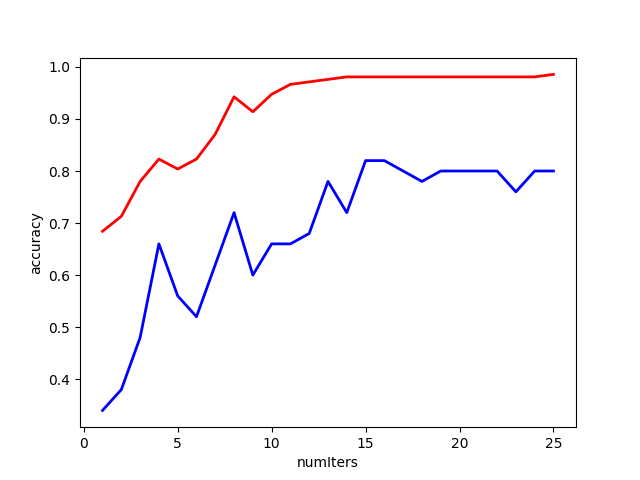【图6】
最后用一个例子来看一下模型的效果——判断一张图片是不是猫：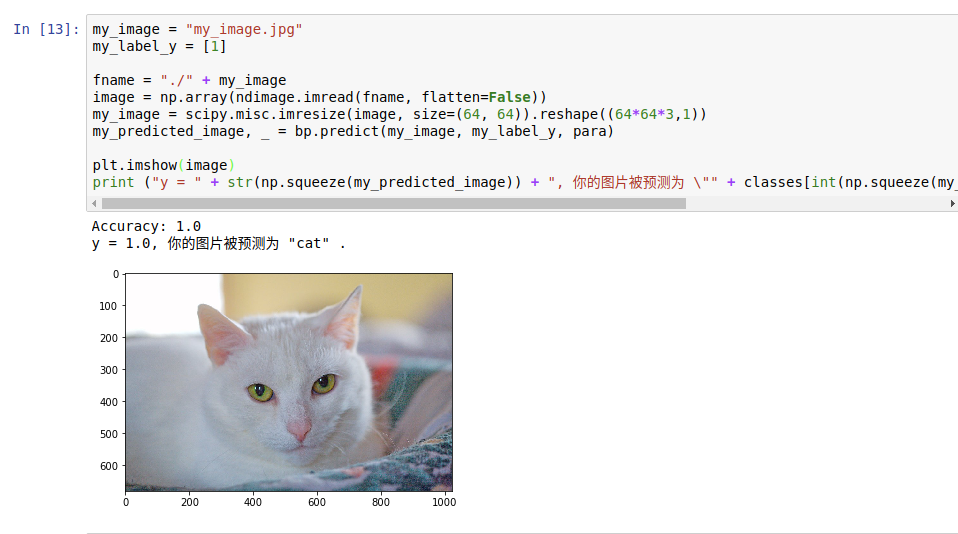【图7】
好了，测试到此结束。你也可以自己尝试其它的神经网络结构和测试其它图片。
5. 本文小结
本文主要叙述了经典的全连接神经网络结构以及前向传播和反向传播的过程。通过本文的学习，读者应该可以独立推导全连接神经网络的传播过程，对算法的细节烂熟于心。另外，由于本文里的公式大部分是我自己推导的，瑕疵之处，希望读者不吝赐教。
虽然这篇文章实现的例子并没有什么实际应用场景，但是自己推导一下这些数学公式并用代码实现对理解神经网络内部的原理很有帮助，继这篇博客之后，我还计划写一个如何自己推导并实现卷积神经网络的教程，如果有人感兴趣，请继续关注我！
本次内容就到这里，谢谢大家。
订正与答疑：
前向传播过程比较简单，我就不再赘述了。
这里主要针对反向传播过程中可能会出现的问题做一个总结：
1. 具体解释一下公式1里面的“堆砌”是什么意思？

δ

j

[

L

]

=

∑

k

∂

L

∂

a

k

[

L

]

∂

a

k

[

L

]

∂

z

j

[

L

]

\delta^{[L]}_j = \sum_k \frac{\partial L}{\partial a^{[L]}_k} \frac{\partial a^{[L]}_k}{\partial z^{[L]}_j}

有读者对这里不太理解，这其实是因为，我们的输出层不一定是只有一个神经元，可能有好多个神经元，因此损失函数是每个输出神经元“误差”之和，因此才会出现这种

∑

\sum

的形式，然后每个输出神经元的误差函数与其它神经元没有关系，所以只有

k

=

j

k=j

的时候值不是0.
另外，这里说的“堆砌”指的就是：

δ

[

l

]

=

[

∂

L

∂

a

1

[

L

]

∂

L

∂

a

2

[

L

]

⋮

]

⊙

[

σ

′

(

z

1

[

L

]

)

σ

′

(

z

2

[

L

]

)

⋮

]

\delta^{[l]}=\left[ \begin{array}{cc} \frac{\partial L}{\partial a^{[L]}_1} \\ \frac{\partial L}{\partial a^{[L]}_2} \\\vdots \end{array}\right]\odot\left[\begin{array}{cc}\sigma^{&#x27;}(z^{[L]}_1) \\\sigma^{&#x27;}(z^{[L]}_2)\\\vdots\end{array}\right]

2. 公式2写成矩阵形式为什么系数矩阵会有转置？自己没搞懂。
这里可能有一点绕，有的读者感觉我的推导不是很明白，所以有必要详细说明一下。
很多读者不明白，写成矩阵形式的时候

δ

[

l

]

=

[

w

[

l

+

1

]

T

δ

[

l

+

1

]

]

⊙

σ

′

(

z

[

l

]

)

\delta^{[l]}=[w^{[l+1]T}\delta^{[l+1]}]\odot \sigma ^{&#x27;}(z^{[l]})

里面的“系数矩阵转置”是怎么来的。这里就主要说明一下：
相信大家都已经理解了下面这个前向传播公式：

z

k

[

l

+

1

]

=

∑

j

w

k

j

[

l

+

1

]

a

j

[

l

]

+

b

k

[

l

+

1

]

=

∑

j

w

k

j

[

l

+

1

]

σ

(

z

j

[

l

]

)

+

b

k

[

l

+

1

]

z^{[l+1]}_k=\sum_jw^{[l+1]}_{kj}a^{[l]}_j+b^{[l+1]}_k=\sum_jw^{[l+1]}_{kj}\sigma(z^{[l]}_j)+b^{[l+1]}_k

求偏导这里在原文中有一点错误，应该是：

∂

z

k

[

l

+

1

]

∂

z

j

[

l

]

=

∑

k

w

k

j

[

l

+

1

]

σ

′

(

z

j

[

l

]

)

\frac{\partial z^{[l+1]}_k}{\partial z^{[l]}_j} =\sum_k w^{[l+1]}_{kj} \sigma&#x27;(z^{[l]}_j)

为了大家有一个直观的感受，来一个具体的例子：
第 1 层的系数矩阵比方是：

w

[

2

=

[

w

11

[

2

]

w

12

[

2

]

w

13

[

2

]

w

21

[

2

]

w

22

[

2

]

w

23

[

2

]

]

w^{[2}=\left[ \begin{array}{cc} w_{11}^{} &amp; w_{12}^{} &amp; w_{13}^{} \\ w_{21}^{}&amp; w_{22}^{} &amp; w_{23}^{}\end{array}\right]

b

[

2

]

=

[

b

1

[

2

]

b

2

[

2

]

b^{}=\left[ \begin{array}{cc}b^{}_1 \\ b^{[2}_2 \end{array}\right]

z

[

2

]

=

[

w

11

[

2

]

w

12

[

2

]

w

13

[

2

]

w

21

[

2

]

w

22

[

2

]

w

23

[

2

]

]

⋅

[

a

1

[

1

]

a

2

[

1

]

a

3

[

1

]

]

+

[

b

1

[

2

]

b

2

[

2

]

]

=

[

w

11

[

2

]

a

1

[

1

]

+

w

12

[

2

]

a

2

[

1

]

+

w

13

[

2

]

a

3

[

1

]

+

b

1

[

2

]

w

21

[

2

]

a

1

[

1

]

+

w

22

[

2

]

a

2

[

1

]

+

w

23

[

2

]

a

3

[

1

]

+

b

2

[

2

]

]

z^{}=\left[ \begin{array}{cc} w_{11}^{} &amp; w_{12}^{} &amp; w_{13}^{} \\ w_{21}^{}&amp; w_{22}^{} &amp; w_{23}^{}\end{array}\right]\cdot \left[ \begin{array}{cc} a^{}_1 \\ a^{}_2 \\ a^{}_3 \end{array}\right] +\left[ \begin{array}{cc}b^{}_1 \\ b^{}_2 \end{array}\right]=\left[ \begin{array}{cc} w_{11}^{}a^{}_1+w_{12}^{}a^{}_2+w_{13}^{}a^{}_3+b^{}_1 \\ w^{}_{21}a^{}_1+w_{22}^{}a^{}_2+w_{23}^{}a^{}_3+b^{}_2\end{array}\right]

那么，

∂

z

1

[

2

]

∂

a

1

[

1

]

=

∂

(

w

11

[

2

]

a

1

[

1

]

+

w

12

[

2

]

a

2

[

1

]

+

w

13

[

2

]

a

3

[

1

]

+

b

1

[

2

]

)

∂

a

1

[

1

]

=

w

11

[

2

]

\frac{\partial z^{}_1}{\partial a^{}_1}=\frac{\partial(w_{11}^{}a^{}_1+w_{12}^{}a^{}_2+w_{13}^{}a^{}_3+b^{}_1)}{\partial a^{}_1}=w^{}_{11}

那么，根据之前介绍的求解梯度向量的定义：

∂

z

1

[

2

]

∂

a

[

1

]

=

[

∂

z

1

[

2

]

∂

a

1

[

1

]

∂

z

1

[

2

]

∂

a

2

[

1

]

∂

z

1

[

2

]

∂

a

3

[

1

]

]

=

[

w

11

[

2

]

w

12

[

2

]

w

13

[

2

]

]

\frac{\partial z^{}_1}{\partial a^{}}=\left[ \begin{array}{cc}\frac{\partial z^{}_1}{\partial a^{}_1}\\\frac{\partial z^{}_1}{\partial a^{}_2}\\\frac{\partial z^{}_1}{\partial a^{}_3}\end{array}\right]=\left[\begin{array}{cc}w^{}_{11}\\w^{}_{12}\\w^{}_{13}\end{array}\right]

∂

z

2

[

2

]

∂

a

[

1

]

=

[

∂

z

2

[

2

]

∂

a

1

[

1

]

∂

z

2

[

2

]

∂

a

2

[

1

]

∂

z

2

[

2

]

∂

a

3

[

1

]

]

=

[

w

21

[

2

]

w

22

[

2

]

w

23

[

2

]

]

\frac{\partial z^{}_2}{\partial a^{}}=\left[ \begin{array}{cc}\frac{\partial z^{}_2}{\partial a^{}_1}\\\frac{\partial z^{}_2}{\partial a^{}_2}\\\frac{\partial z^{}_2}{\partial a^{}_3}\end{array}\right]=\left[\begin{array}{cc}w^{}_{21}\\w^{}_{22}\\w^{}_{23}\end{array}\right]